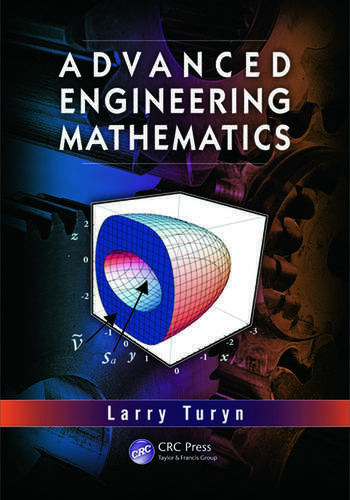## 1st Edition

Lawrence Turyn

CRC Press
Published September 25, 2013
Textbook - 1455 Pages - 353 B/W Illustrations
ISBN 9781439834473 - CAT# K11552

For Instructors Request Inspection Copy

was \$215.00

USD\$172.00

SAVE ~\$43.00

FREE Standard Shipping!

## Preview

### Summary

Beginning with linear algebra and later expanding into calculus of variations, Advanced Engineering Mathematics provides accessible and comprehensive mathematical preparation for advanced undergraduate and beginning graduate students taking engineering courses. This book offers a review of standard mathematics coursework while effectively integrating science and engineering throughout the text. It explores the use of engineering applications, carefully explains links to engineering practice, and introduces the mathematical tools required for understanding and utilizing software packages.

• Provides comprehensive coverage of mathematics used by engineering students
• Combines stimulating examples with formal exposition and provides context for the mathematics presented
• Contains a wide variety of applications and homework problems
• Includes over 300 figures, more than 40 tables, and over 1500 equations
• Introduces useful Mathematica™ and MATLAB® procedures
• Presents faculty and student ancillaries, including an online student solutions manual, full solutions manual for instructors, and full-color figure sides for classroom presentations

Advanced Engineering Mathematics covers ordinary and partial differential equations, matrix/linear algebra, Fourier series and transforms, and numerical methods. Examples include the singular value decomposition for matrices, least squares solutions, difference equations, the z-transform, Rayleigh methods for matrices and boundary value problems, the Galerkin method, numerical stability, splines, numerical linear algebra, curvilinear coordinates, calculus of variations, Liapunov functions, controllability, and conformal mapping.

This text also serves as a good reference book for students seeking additional information. It incorporates Short Takes sections, describing more advanced topics to readers, and Learn More about It sections with direct references for readers wanting more in-depth information.

#### Instructors

We provide complimentary e-inspection copies of primary textbooks to instructors considering our books for course adoption.